Courses

Test: Stability

15 Questions MCQ Test RRB JE for Electrical Engineering | Test: Stability

Description
Attempt Test: Stability | 15 questions in 45 minutes | Mock test for Electronics and Communication Engineering (ECE) preparation | Free important questions MCQ to study RRB JE for Electrical Engineering for Electronics and Communication Engineering (ECE) Exam | Download free PDF with solutions
QUESTION: 1

The forward-path transfer function of a ufb system is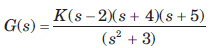For system to be stable, the range of K is

Solution: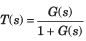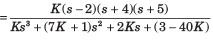Routh table is as shown in fig. S.6.211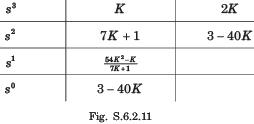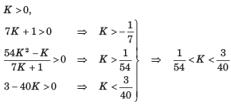QUESTION: 2

Solution:
QUESTION: 3

The open-loop transfer function of a ufb system is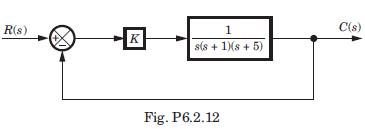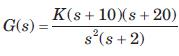The closed loop system will be stable if the value of K is

Solution: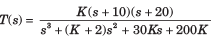Routh table is as shown in fig.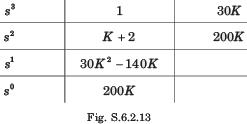200K > 0 → K > 0, 30K2 - 140K > 0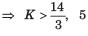satisfy this condition.

QUESTION: 4

The closed loop transfer function for this system is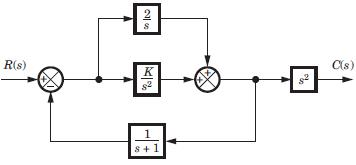Solution:

First combine the parallel loop K/s2
and 2/s giving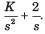Then apply feedback formula with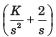and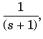and then multiply with s2.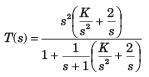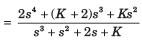QUESTION: 5

The poles location for this system is shown in fig.The value of K is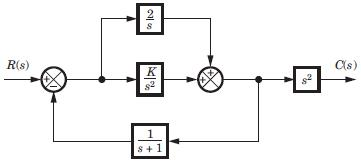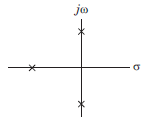Solution:

Denominator = s3 + s2 + 2s + K Routh table is as shown in fig.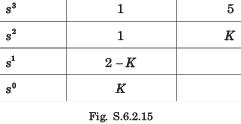Row of zeros when K = 2,
s2 + 2 = 0, ⇒ s = -1, j√2, - j√2

QUESTION: 6

The forward path transfer of ufb system is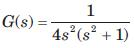The system is

Solution:
QUESTION: 7

The forward-path transfer function of a ufb system is T(s) =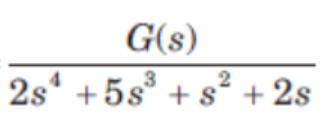The system is

Solution:

Closed loop transfer function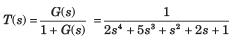Routh table is as shown in fig. S.6.2.28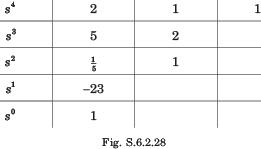2 RHP poles so unstable.

QUESTION: 8

The open loop transfer function of a system is as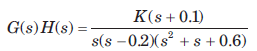The range of K for stable system will be

Solution:

The characteristic equation is 1 + G(s)H(s) = 0

⇒ s(s - 0.2)(s2 + s + 0.6)+K(s + 0.1) = 0
s4 +0.8 s3 +0.4s2 +(K - 0.12)s +0.1K = 0
Routh table is as shown in fig. S.62.29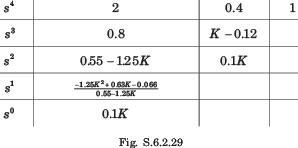K > 0, 055 -125K > 0 ⇒ K < 0.44 -125K2 +0.63K -0066 >0
(K - 0.149)(K - 0355) < 0, 0.149 < K < 0.355

QUESTION: 9

The open-loop transfer function of a ufb control system is given by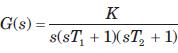For the system to be stable the range of K is

Solution:

Characteristic equation

s(sT1 + 1)(sT2 +1) + K = 0
T1T2s3 + (T1 + T2)s2 + s + K = 0
Routh table is as shown in fig S.6.2.30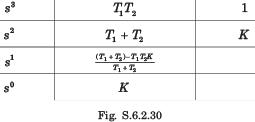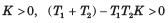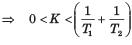QUESTION: 10

If the roots of the have negative real parts, then the response is ____________

Solution:

If the roots of the have negative real parts then the response is bounded and eventually decreases to zero.

QUESTION: 11

The closed loop transfer function of a system is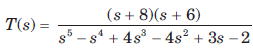The number of poles in RHP and in LHP are

Solution: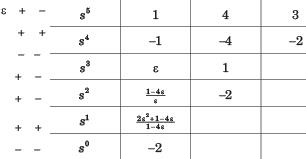3 RHP, 2 LHP poles.

QUESTION: 12

The closed loop transfer function of a system is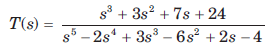The number of poles in LHP, in RHP, and on jω - axis are

Solution: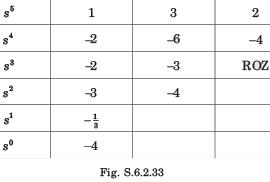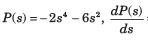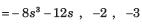No sign change exist from the s4 row down to the s0 row.
Thus, the even polynomial does not have RHP poles. Therefore because of symmetry all four poles must be on jw -axis.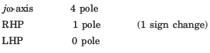QUESTION: 13

For the system shown in fig. the number of poles on RHP, LHP, and imaginary axis are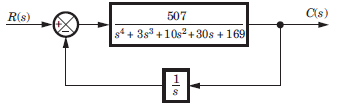Solution:

Closed loop transfer function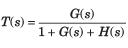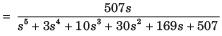Routh table is as shown in fig. S.6.2.34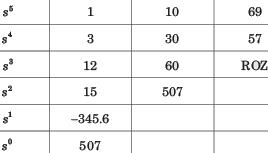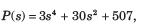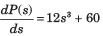From s4 row down to s0 there is one sign change. So LHP–1 + 1= 2 pole. RHP–1 pole, jw - axis - 2 pole.

QUESTION: 14

If a system is given unbounded input then the system is:

Solution:

If the system is given with the unbounded input then nothing can be clarified for the stability of the system.

QUESTION: 15

For the open loop system of fig. location of poles on RHP, LHP, and an jω - axis are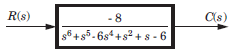Solution:

Routh table is as shown in fig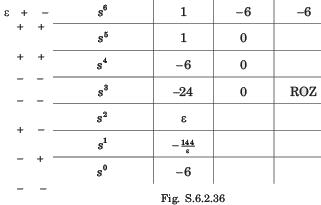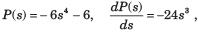Them is two sign change from the s4 mw down to the s° row. So two roots are on RHS. Because of symmetry rest two roots must be in LHP. From s6 to s4 there is 1 sign change so 1 on RHP and 1 on LHP.
Total LHP 3 root, RHP 3 root.Use Code STAYHOME200 and get INR 200 additional OFF Use Coupon Code

Track your progress, build streaks, highlight & save important lessons and more!

Similar ContentRelated tests